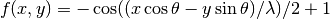# utilities – Helper functions¶

The utilities package is the standard home for shared functions which many modules may use. The spirit of utilities is also to isolate sections of critical code so that unit tests can be used to ensure a minimum of bugginess.

## Coordinate Handling¶

bravo.utilities.coords.split_coords(x, z)[source]

Split a pair of coordinates into chunk and subchunk coordinates.

Parameters: x (int) – the X coordinate z (int) – the Z coordinate a tuple of the X chunk, X subchunk, Z chunk, and Z subchunk

## Data Packing¶

More affectionately known as “bit-twiddling.”

## Trigonometry¶

bravo.utilities.maths.rotated_cosine(x, y, theta, lambd)[source]

Evaluate a rotated 3D sinusoidal wave at a given point, angle, and wavelength.

The function used is:This function has a handful of useful properties; it has a local minimum at f(0, 0) and oscillates infinitely betwen 0 and 1.

Parameters: x (float) – X coordinate y (float) – Y coordinate theta (float) – angle of rotation lambda (float) – wavelength float of f(x, y)

### Table Of Contents

#### Previous topic

simplex – Simplex noise generation

Tools